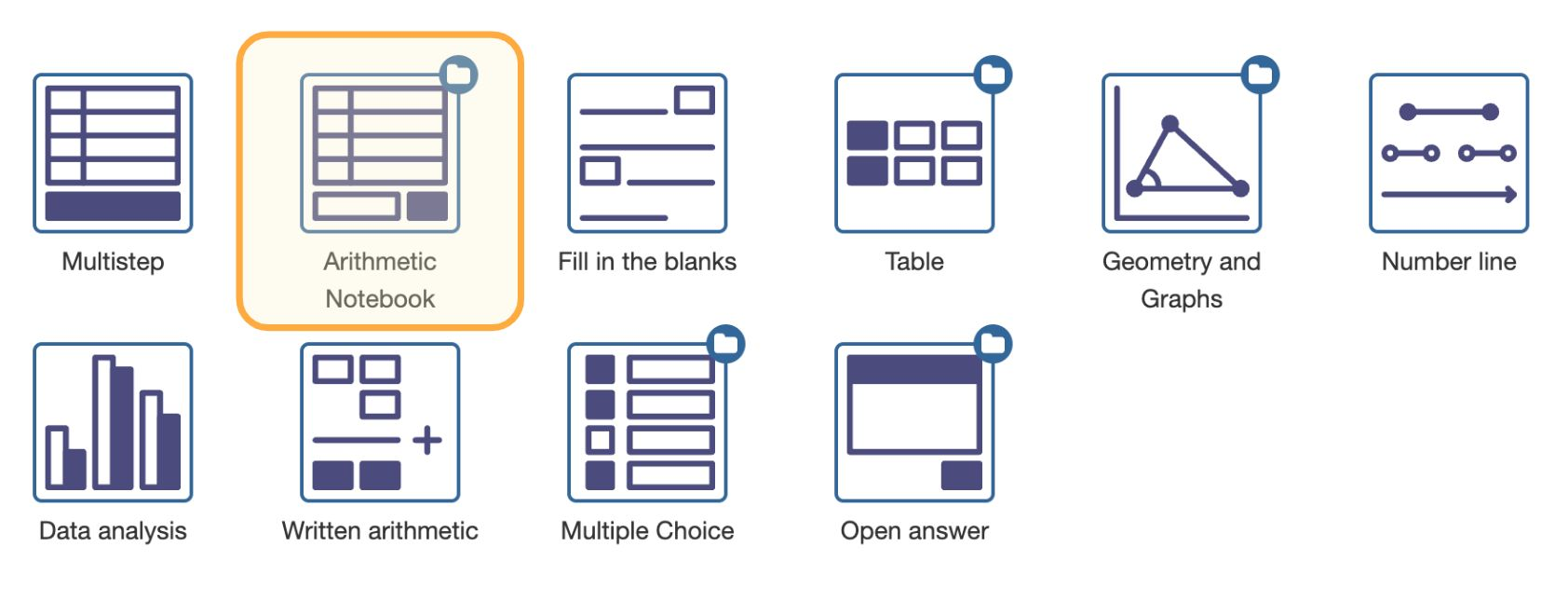# Arithmetic NotebookFigure: Selecting the Arithmetic Notebook question type.

Arithmetic Notebook is a question type for arithmetic story problems. These are story problems that do not require variables or formulas but only a calculator.

The goal of story problems is to learn to apply mathematics to solve real-world problems. Students need to accomplish several tasks when working on story problems, such as:

1. Decomposing the problem into partial problems
2. Writing a mathematical formulation of each partial problem
3. Using math skills or a calculator to solve the mathematical problems
4. Interpreting the math results to formulate the answer to the real-world problem.

Arithmetic Notebook is designed to challenge students on all these steps.

If you have already read about Multistep's support for arithmetic story problems, you will discover Arithmetic Notebook and Multistep have a lot in common. Creating exercises is very similar for both question types. However, we will not assume you already know about Multistep and repeat concepts that you need to learn to create content with Arithmetic Notebook.